# polarpattern

Interactive plot of radiation patterns in polar format

## Description

The `polarpattern` object creates an interactive plot of antenna or array radiation patterns in polar format with uniformly spaced angles. You can also plot other types of polar data. Use this plot for interactive data visualization or measurement. To change the properties, zoom in, or add more data to the plot, right-click or scroll or drag the Polar Measurement window.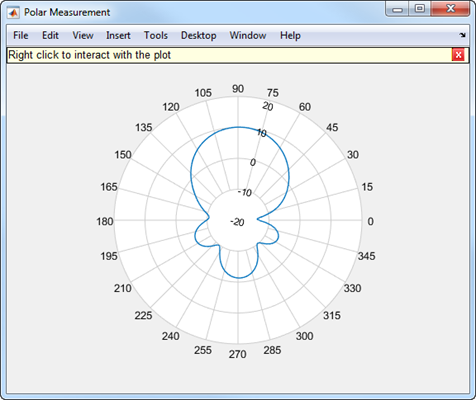## Creation

### Syntax

``polarpattern``
``polarpattern(data)``
``polarpattern(angle,magnitude)``
``polarpattern(___,Name=Value)``
``polarpattern(ax,___)``
``p = polarpattern(___)``
``p = polarpattern('gco')``

### Description

````polarpattern` creates an empty polar plot. You can add plots of antenna or array radiation patterns and other types of data to the plot by importing saved polari objects from MAT-files.```

example

````polarpattern(data)` creates a polar plot with real magnitude values in the vector `data` with angles uniformly spaced on the unit circle starting at `0` degrees. Magnitudes may be negative when dB data units are used. For a matrix `data`, columns of `data` are independent datasets. For N-`data` arrays, dimensions 2 and greater are independent datasets. For complex values, magnitude and angle are derived from `data`.```

example

````polarpattern(angle,magnitude)` creates a polar plot for a set of angles and corresponding magnitudes. You can also create polar plots from multiple sets of angle vectors in degrees and corresponding sets of magnitude using the syntax: ```polarpattern(angle1, magnitude1,..., angleN, magnitudeN)```.```

example

````polarpattern(___,Name=Value)` creates a polar plot, with additional PolarPattern Properties specified by one or more name-value pair arguments. `Name` is the property name and `Value` is the corresponding property value. You can specify several name-value pair arguments in any order as `Name1`=`Value1`, `...`, `NameN`=`ValueN`. Unspecified properties retain their default values. To list all the property names and values, use `details(p)`. You can use the properties to extract data about the radiation pattern from the polar plot. For example, `p = polarpattern(data,Peaks=3)` identifies and displays the three highest peaks in the pattern data.```
````polarpattern(ax,___)` creates a polar plot using axes object, `ax` instead of the current axes object.```
````p = polarpattern(___)` creates a polari object using any combination of input arguments from the previous syntaxes. Use this syntax to customize the plot or add measurements.```
````p = polarpattern('gco')` creates a polar plot object from the polar pattern in the current figure.```

### Input Arguments

expand all

Antenna or array data, specified as one of these options

• A real length-M vector, containing M magnitude values with angles their defined as $\frac{\left(0:M-1\right)}{M}×{360}^{\circ }$ degrees.

• A real M-by-N matrix, containing M magnitude values in a dataset and N such independent data sets. Each column of the matrix has angles in degrees from the vector $\frac{\left(0:M-1\right)}{M}×{360}^{\circ }$.

• A real multidimensional array. Arrays with `2` or more dimensions contain independent data sets.

• A complex vector or matrix, that contains Cartesian coordinates (x, y) of each point. x contains the real part of the `data` and y contains the imaginary part of the `data`.

When the data is in a logarithmic form, such as dB, magnitude values can be negative. In this case, `polarpattern` plots the smallest magnitude values at the origin of the polar plot and largest magnitude values at the maximum radius.

Data Types: `double`
Complex Number Support: Yes

Set of angles in degrees, specified as a vector.

Data Types: `double`

Set of magnitude values, specified as a vector or a matrix. If you specify this input as a matrix, each column is an independent set of magnitude values and corresponds to the same set of angles in the same column of the angle input.

Data Types: `double`

Axes of the polar plot, specified as an axes object.

### Output Arguments

expand all

Stores a polari object with a set of properties. Use p to modify properties of the plot after creation. For a list of all the properties, see PolarPattern Properties.

Example: `P = polarplot(V)`

## Object Functions

 `add` Add data to polar plot `addCursor` Add cursor to polar plot angle `animate` Replace existing data with new data for animation `createLabels` Create legend labels for polar plot `findLobes` Main, back, and side lobe data `replace` Replace polar plot data with new data `showPeaksTable` Show or hide peak marker table `showSpan` Show or hide angle span between two markers

## Examples

collapse all

Create a default Vivaldi antenna and calculate the directivity at 1.5 GHz.

```v = vivaldi; V = pattern(v,1.5e9,0,0:1:360);```

Plot the polar pattern of the calculated directivity.

`P = polarpattern(V);`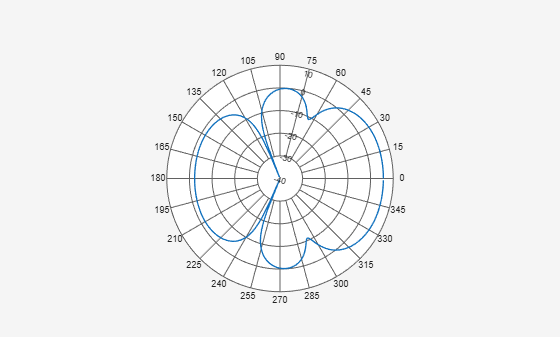Create a default cavity antenna. Calculate the directivity of the antenna and write the data to `cavity.pln` using the `msiwrite` function.

```c = cavity; msiwrite(c,2.8e9,"cavity",Name="Cavity Antenna Specifications");```

Read the cavity specification file into `Horizontal`, `Vertical`, and `Optional` structures using the `msiread` function.

`[Horizontal,Vertical,Optional] = msiread("cavity.pln")`
```Horizontal = struct with fields: PhysicalQuantity: 'Gain' Magnitude: [360x1 double] Units: 'dBi' Azimuth: [360x1 double] Elevation: 0 Frequency: 2.8000e+09 Slice: 'Elevation' ```
```Vertical = struct with fields: PhysicalQuantity: 'Gain' Magnitude: [360x1 double] Units: 'dBi' Azimuth: 0 Elevation: [360x1 double] Frequency: 2.8000e+09 Slice: 'Azimuth' ```
```Optional = struct with fields: name: 'Cavity Antenna Specifications' frequency: 2.8000e+09 gain: [1x1 struct] ```

Plot the polar pattern of the cavity at azimuth angles.

`P = polarpattern(Horizontal.Azimuth,Horizontal.Magnitude);`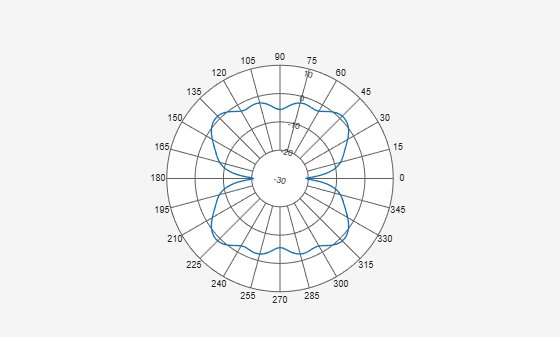Create a default monopole antenna and calculate the directivity at 75 MHz.

```m = monopole; M = pattern(m,75e6,0,0:1:360);```

Plot the polar pattern of the antenna.

`P = polarpattern(M,TitleTop="Polar Pattern of Monopole");`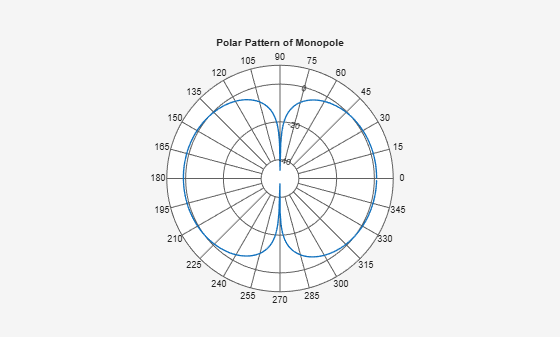Plot the radiation pattern of a dipole antenna operating at 75 MHz. using `polarpattern` and specify a title to the plot. Then, display the `polarpattern` properties.

Create a default dipole antenna.

```d = dipole; fc = 75e6;```

Calculate its directivity at 75 MHz. and plot the polar pattern for an elevation cut at 0° azimuth.

```D = pattern(d,fc,0,0:1:360); P = polarpattern(D,TitleTopTextInterpreter="tex",... TitleTop="Elevation Cut (Azimuth Angle = 0^{\circ})");```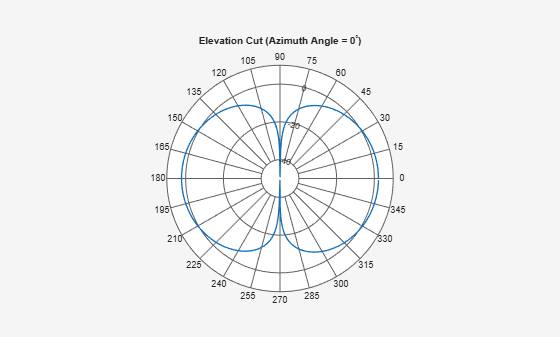Display the properties of the plot.

`details(P)`
``` internal.polari handle with properties: Interactive: 1 LegendLabels: '' AntennaMetrics: 0 CleanData: 1 AngleData: [361x1 double] MagnitudeData: [361x1 double] IntensityData: [] AngleMarkers: [0x1 struct] CursorMarkers: [0x1 struct] PeakMarkers: [0x1 struct] ActiveDataset: 1 AngleLimVisible: 0 LegendVisible: 0 Span: 0 TitleTop: 'Elevation Cut (Azimuth Angle = 0^{\circ})' TitleBottom: '' Peaks: [] FontSize: 10 MagnitudeLim: [-50 10] MagnitudeAxisAngle: 75 MagnitudeTick: [-40 -20 0] MagnitudeTickLabelColor: 'k' AngleLim: [0 360] AngleTickLabel: {'0' '15' '30' '45' '60' '75' '90' '105' '120' '135' '150' '165' '180' '195' '210' '225' '240' '255' '270' '285' '300' '315' '330' '345'} AngleTickLabelColor: 'k' TitleTopFontSizeMultiplier: 1.1000 TitleBottomFontSizeMultiplier: 0.9000 TitleTopFontWeight: 'bold' TitleBottomFontWeight: 'normal' TitleTopTextInterpreter: 'tex' TitleBottomTextInterpreter: 'none' TitleTopOffset: 0.1500 TitleBottomOffset: 0.1500 ToolTips: 1 MagnitudeLimBounds: [-Inf Inf] MagnitudeFontSizeMultiplier: 0.9000 AngleFontSizeMultiplier: 1 AngleAtTop: 90 AngleDirection: 'ccw' AngleResolution: 15 AngleTickLabelRotation: 0 AngleTickLabelFormat: '360' AngleTickLabelColorMode: 'contrast' PeaksOptions: {} AngleTickLabelVisible: 1 Style: 'line' DataUnits: 'dB' DisplayUnits: 'dB' NormalizeData: 0 ConnectEndpoints: 0 DisconnectAngleGaps: 0 EdgeColor: 'k' LineStyle: '-' LineWidth: 1 FontName: 'Helvetica' FontSizeMode: 'auto' GridForegroundColor: [0.8000 0.8000 0.8000] GridBackgroundColor: 'w' DrawGridToOrigin: 0 GridOverData: 0 GridAutoRefinement: 0 GridWidth: 0.5000 GridVisible: 1 ClipData: 1 TemporaryCursor: 1 MagnitudeLimMode: 'auto' MagnitudeAxisAngleMode: 'auto' MagnitudeTickMode: 'auto' MagnitudeTickLabelColorMode: 'contrast' MagnitudeTickLabelVisible: 1 MagnitudeUnits: '' IntensityUnits: '' Marker: 'none' MarkerSize: 6 Parent: [1x1 Figure] NextPlot: 'replace' ColorOrder: [7x3 double] ColorOrderIndex: 1 SectorsColor: [16x3 double] SectorsAlpha: 0.5000 View: 'full' ZeroAngleLine: 0 ```

Remove `-inf` and `NaN` values from monopole antenna polar pattern data by using the `CleanData` and `AntennaMetrics` properties of a polari object. Use `CleanData` for partial data with `-inf` and `NaN` values.

```m = monopole; m.GroundPlaneLength = inf;```

Plot the beamwidth of the antenna at 70 MHz.

```figure; beamwidth(m,70e6,0,-50:30)```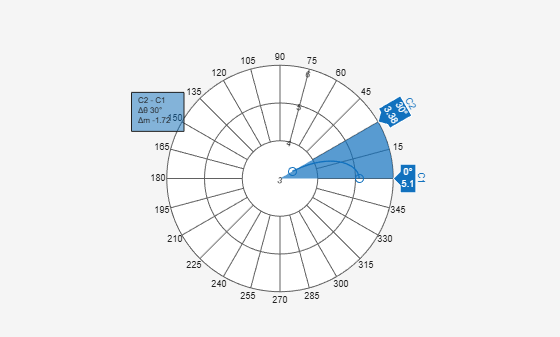Plot the radiation pattern of the antenna at 70 MHz.

```figure; pattern(m,70e6,0,-50:30);```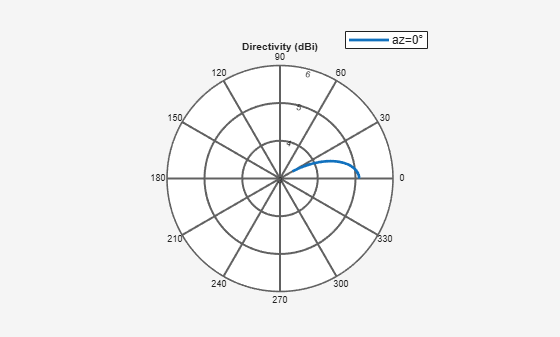Use `polarpattern` to view the antenna metrics of the radiation pattern.

```P = polarpattern('gco'); P.CleanData = 1; P.AntennaMetrics = 1;```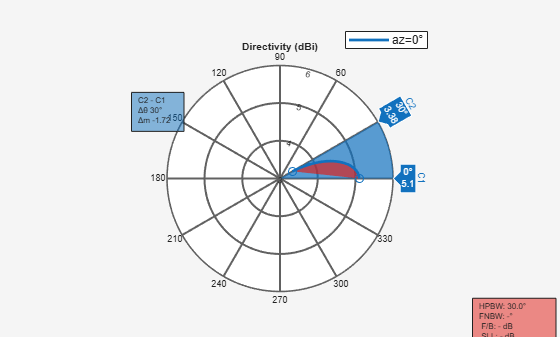Compare the `beamwidth` plot and the `polarpattern` plot. The Antenna Metrics does not represent the beamwidth correctly.

You can also clean the data by right clicking on the plot and selecting Clean Data.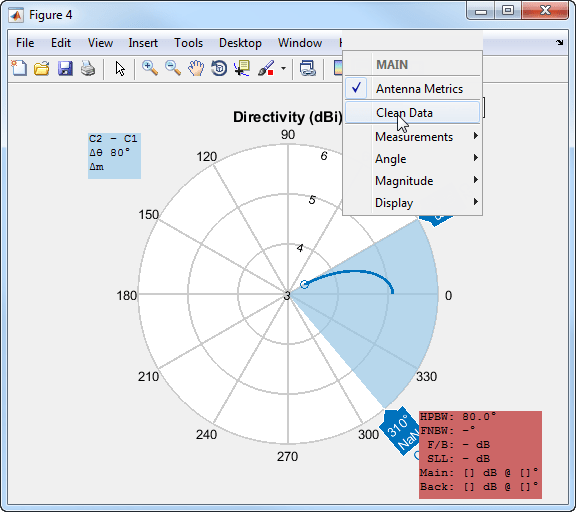After you clean the data, the `polarpattern` plot calculation matches the `beamwidth` plot calculation.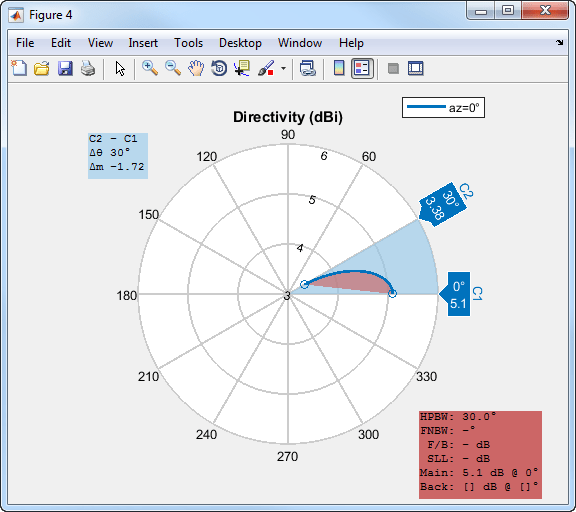## Version History

Introduced in R2016a